Newton's Laws - Lesson 1 - Newton's First Law of Motion

# Balanced and Unbalanced Forces

Newton's first law of motion has been frequently stated throughout this lesson.

An object at rest stays at rest and an object in motion stays in motion with the same speed and in the same direction unless acted upon by an unbalanced force.

###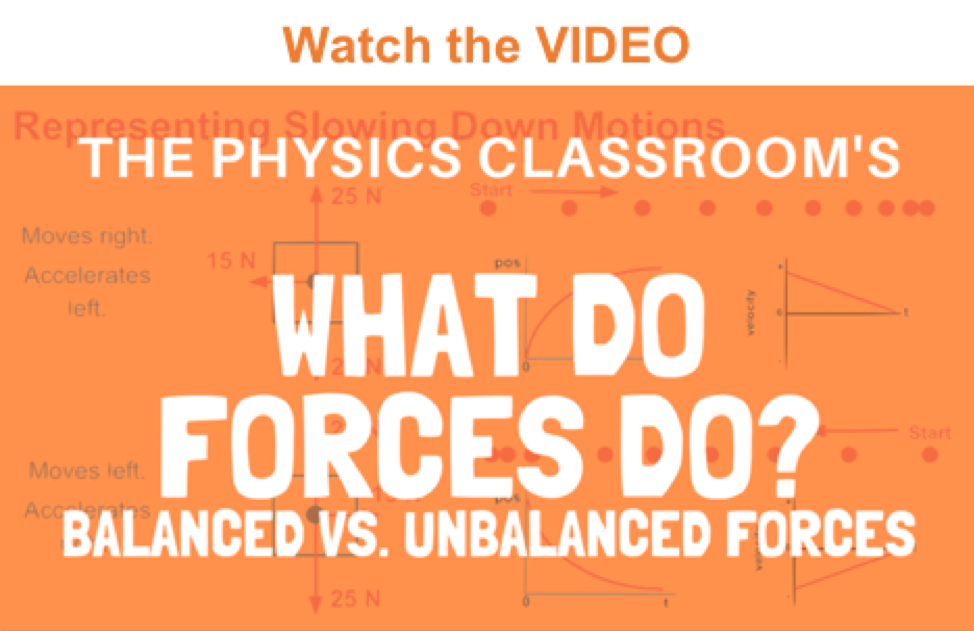Balanced Forces

But what exactly is meant by the phrase unbalanced force? What is an unbalanced force? In pursuit of an answer, we will first consider a physics book at rest on a tabletop. There are two forces acting upon the book. One force - the Earth's gravitational pull - exerts a downward force. The other force - the push of the table on the book (sometimes referred to as a normal force) - pushes upward on the book.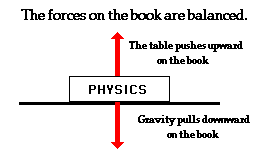Since these two forces are of equal magnitude and in opposite directions, they balance each other. The book is said to be at equilibrium. There is no unbalanced force acting upon the book and thus the book maintains its state of motion. When all the forces acting upon an object balance each other, the object will be at equilibrium; it will not accelerate. (Note: diagrams such as the one above are known as free-body diagrams and will be discussed in detail in Lesson 2.)

Consider another example involving balanced forces - a person standing on the floor. There are two forces acting upon the person. The force of gravity exerts a downward force. The floor exerts an upward force.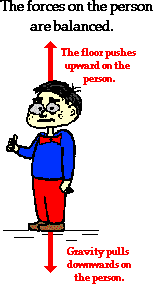Since these two forces are of equal magnitude and in opposite directions, they balance each other. The person is at equilibrium. There is no unbalanced force acting upon the person and thus the person maintains its state of motion. (Note: diagrams such as the one above are known as free-body diagrams and will be discussed in detail in Lesson 2.)

###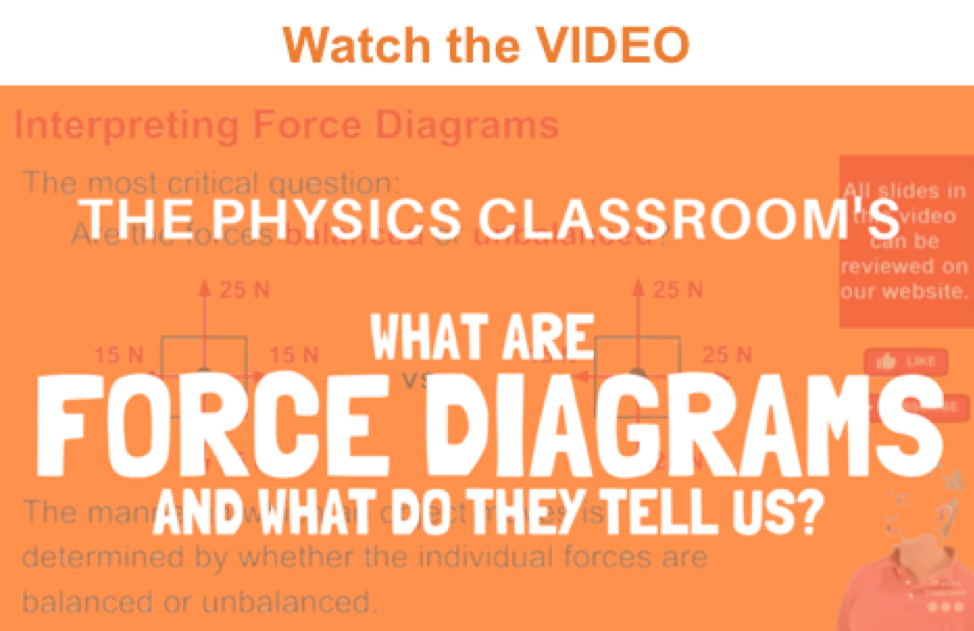Unbalanced Forces

Now consider a book sliding from left to right across a tabletop. Sometime in the prior history of the book, it may have been given a shove and set in motion from a rest position. Or perhaps it acquired its motion by sliding down an incline from an elevated position. Whatever the case, our focus is not upon the history of the book but rather upon the current situation of a book sliding to the right across a tabletop. The book is in motion and at the moment there is no one pushing it to the right. (Remember: a force is not needed to keep a moving object moving to the right.) The forces acting upon the book are shown below.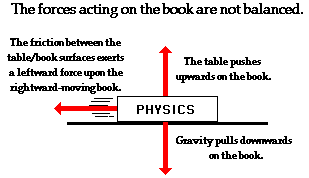The force of gravity pulling downward and the force of the table pushing upwards on the book are of equal magnitude and opposite directions. These two forces balance each other. Yet there is no force present to balance the force of friction. As the book moves to the right, friction acts to the left to slow the book down. There is an unbalanced force; and as such, the book changes its state of motion. The book is not at equilibrium and subsequently accelerates. Unbalanced forces cause accelerations. In this case, the unbalanced force is directed opposite the book's motion and will cause it to slow down. (Note: diagrams such as the one above are known as free-body diagrams and will be discussed in detail in Lesson 2.)

To determine if the forces acting upon an object are balanced or unbalanced, an analysis must first be conducted to determine what forces are acting upon the object and in what direction. If two individual forces are of equal magnitude and opposite direction, then the forces are said to be balanced. An object is said to be acted upon by an unbalanced force only when there is an individual force that is not being balanced by a force of equal magnitude and in the opposite direction. Such analyses are discussed in Lesson 2 of this unit and applied in Lesson 3.

### We Would Like to Suggest ...Sometimes it isn't enough to just read about it. You have to interact with it! And that's exactly what you do when you use one of The Physics Classroom's Interactives. We would like to suggest that you combine the reading of this page with the use of our Rocket Sledder Interactive. You can find it in the Physics Interactives section of our website. The Rocket Sledder allows a learner to explore the effect of balanced and unbalanced forces upon the acceleration of a rocket-propelled sledder.

Visit:  Rocket Sledder

Luke Autbeloe drops an approximately 5.0 kg box of shingles (weight = 50.0 N) off the roof of his house into the swimming pool below. Upon encountering the pool, the box encounters a 50.0 N upward resistance force (assumed to be constant). Use this description to answer the following questions. Click the button to view the correct answers.

1. Which one of the velocity-time graphs best describes the motion of the box? Support your answer with sound reasoning.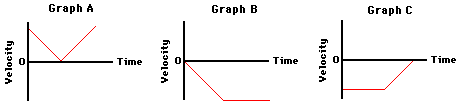2. Which one of the following dot diagrams best describes the motion of the falling box from the time that they are dropped to the time that they hit the bottom of the pool? The arrows on the diagram represent the point at which the box hits the water. Support your answer with sound reasoning.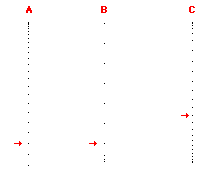3. Several of Luke's friends were watching the motion of the falling box. Being "physics types", they began discussing the motion and made the following comments. Indicate whether each of the comments is correct or incorrect? Support your answers.

a. Once the box hits the water, the forces are balanced and the box will stop.

b. Upon hitting the water, the box will accelerate upwards because the water applies an upward force.

c. Upon hitting the water, the box will bounce upwards due to the upward force.

4. If the forces acting upon an object are balanced, then the object

a. must not be moving.

b. must be moving with a constant velocity.

c. must not be accelerating.

d. none of these